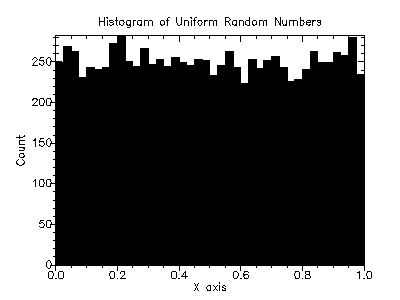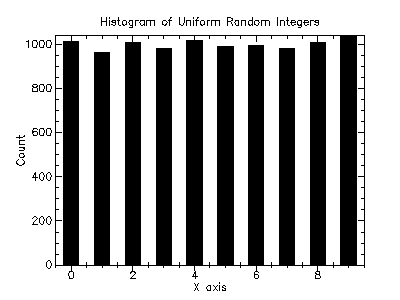Version: 4.2.1

### 6Random Number Generation

The PLT Scheme Science Collection provides additional functionality to the PLT Scheme implementation of SRFI 27 by Sabastian Egner, which, in turn, is a 54-bit implementation ofPierre L’Ecuyer’s MRG32k3a pseudo-random number generator.

The functions described in this chapter are defined in the "random-source.ss" file in the science collection and are made available using the form:

 (require (planet williams/science/random-source))

#### 6.1The SRFI 27 Specification

The following functions are defined in the SRFI specification and are, in purn, provided by the random-source module. The contract shows here are for documentation purposes only–the PLT Scheme implementation of SRFI does not define contracts for its functions.

 (random-integer n) → natural-number/c n : (integer-in 1 +inf.0)
Returns the next integer in {0, ..., n - 1} obtained from the default-random-source. Subsequent results of this function appear to be independent uniformly distributed over the range {0, ..., n - 1}. The argument n must be a positive integer, ptherwise an error is signaled.

 (random-real) → (real-in 0.0 1.0)
Returns the next number, x, 0 < x < 1, obtained from default-random-source. Subsequent results of this procedure appear to be independently uniformly distributed.

 default-random-source
Defines the random source from which random-integer and random-real have been derived using random-source-make-integers and random-source-make-reals. Not that an assignment to default-random-source does not change the behavior of random-real or random-integer; it is strongly recommended not to assign a new value to default-random-source.

 (make-random-source) → random-source?
Creates a new random source. A random source created with make-random-source represents a deterministic stream of random bits. Each random stream obtained as make-random-source generates the same stream of values unless the state is modified with one of the functions below.

 (random-source? x) → boolean? x : any/c
Returns true, #t, if x is a random source, otherwise false, #f, is returned.

 (random-source-state-ref s) → any s : random-source? (random-source-state-set! s state) → any s : random-source? state : any/c
Get and set the current state of the random source s. The implementation of the internal state of a random source is not defined.

 (random-source-randomize! s) → any s : random-state?
Makes an effort to set the state of the random source s to a truly random state.

 (random-source-pseudo-randomize! s i j) → any s : random-source? i : natural-number/c j : natural-number/c
Changes the state of the random source s into the initial state of the (i, j)th independent random source, where i and j are non-negative integers. This procedure provides a mechanism to obtain a large number of independent random sources, indexed by two integers. In contract to random-source-randomize!, this procedure is entirely deterministic.

 (random-source-make-integers s) → (-> (integer-in 1 +inf.0) natural-number/c) s : random-source
Returns a procedure to generate random integers using the random source s. The resulting argument takes a single argument n, which must be a positive integer, and returns the next independent uniformly distributed integer from the interval {0, ..., n - 1} by advancing the state of the random source s.

 (random-source-make-reals s) → (-> (real-in 0.0 1.0)) s : random-source? (random-source-make-reals s unit) → (-> (real-in 0.0 1.0)) s : random-source? unit : (real-in 0.0 1.0)
Obtains a procedure rand to generate random real numbers 0 < x < 1 using the random source s. The procedure rand is called without arguments.

The optional parameter unit determines the type of numbers being produced by rand and the quantization of the output. unit must be a number such that 0 < unit < 1. The numbers created by rand are of the same numerical type as unit and the potential output values are spaced by at most unit. One can imagine rand to create numbers as x*unit where x is a random integer in {1, ..., floor(1/unit)-1}. Note, however, that this need not be the way the values are actually created and that the actual resolution of rand can be much higher than unit. In case unit is absent it defaults to a reasonably small value (related to the width of the mantissa of an efficient number format).

The PLT Scheme Science Collection provides additional functionality to that provided by SRFI 27.

##### 6.2.1The current-random-source parameter

The main additional functionality is to define a parameter, current-random-source, that provides a separate random source reference for each thread. The default value for this random source reference is default-random-source.

The use of the current-random-source parameter overcomes the difficulty with assignment to default-random-source. However, the routines random-integer and random-real use the default-random-source variable and are unaware of the current-random-source parameter.

 (current-random-source) → random-source? (current-random-source s) → void? s : random-source?
Gets or sets the current random source. A guard procedure ensures that the value of current-random-source is indeed a random source, as determined by random-source?, otherwise a type error is raised.

 (with-random-source s body ...+)
Evaluates the body with current-random-source bound to the random source s.

 (with-new-random-source body ...+)
Evaluates the body with current-random-source bound to a newly created random source.

##### 6.2.2Uniform Random Numbers

The PLT Scheme Science Collection provides alternatives to the random-integer and random-real functions that are aware of the current-random-source parameter. The also provide a more convenient interface than random-source-make-integers and random-source-make-reals.

 (random-uniform-int s n) → natural-number? s : random-source? n : (integer-in 1 +inf.0) (random-uniform-int n) → natural-number? n : (integer-in 1 +inf.0)
Returns the next integer in {0, ..., n - 1} obtained from the random source s or (current-random-source) if s is not specified. Subsequent results of this function appear to be independently uniformly distributed over the range {0, ..., n - 1}. The argument n must be a positive integer.

 (random-uniform s) → (real-in 0.0 1.0) s : random-source? (random-uniform) → (real-in 0.0 1.0)
Returns the next number x, 0 < x < 1, obtained from the random source s or (current-random-source) if s is not specified. Subsequent results of this function appear to be independently uniformly distributed.

##### 6.2.3Miscellaneous Functions

These functions provide an alternative set of functions to get or set the state of a random-state. These functions match the conventions for structures in PLT Scheme.

 (random-source-state s) → any s : random-source?
The same as random-source-state-ref.

 (set-random-source-state! s state) → any s : random-state? state : any/c
The same as random-source-state-set!.

##### 6.2.4Random Source Vectors

These functions provide a convenient method for generating a vector of repeatable random sources.

 (make-random-source-vector n i) → (vectorof random-source?) n : natural-number/c i : natural-number/c (make-random-source-vector n) → (vector-of random-source?) n : natural-number/c
Returns a vector of random sources of length n. If i is provided, the jth random source is initialized using (random-source-pseudo-randomize! s i j). If i is not provided, the ith random source is initialized using (random-source-pseudo-randomize! s i 0). Note that this is not the same as having i default to 0.

#### 6.3Examples

Example: Histogram of uniform random numbers.

 #lang scheme (require (planet williams/science/random-source)) (require (planet williams/science/histogram-with-graphics)) (let ((h (make-histogram-with-ranges-uniform 40 0 1)) (s (make-random-source))) (random-source-randomize! s) (with-random-source s (do ((i 0 (+ i 1))) ((= i 10000) (void)) (histogram-increment! h (random-uniform)))) (histogram-plot h "Histogram of Uniform Random Numbers"))

The following figure shows an example of the resulting histogram.Example: Histogram of uniform random integers.

 #lang scheme (require (planet williams/science/random-source)) (require (planet williams/science/histogram-with-graphics)) (let ((h (make-discrete-histogram)) (s (make-random-source))) (random-source-randomize! s) (with-random-source s (do ((i 0 (+ i 1))) ((= i 10000) (void)) (discrete-histogram-increment! h (random-uniform-int 10)))) (discrete-histogram-plot h "Histogram of Uniform Random Integers"))

The following figure shows an example of the resulting histogram.